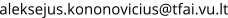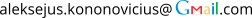# Aleksejus Kononovicius

Senior researcher at Institute of Theoretical Physics and Astronomy (Faculty of Physics, Vilnius University). Research interests: stochastic and agent-based modeling, complex systems, Econophysics, Sociophysics, Statistical Physics and Data Science. Contributor to Physics of Risk science blog.

## Highlights

Intro: My name is Aleksejus Kononovicius (lt. Kononovičius). I hold PhD in Physics and currently work as a senior researcher at Institute of Theoretical Physics and Astronomy (Faculty of Physics, Vilnius University). Besides conducting research in Econophysics and Sociophysics, which involves stochastic and agent-based modeling as well as a bit of Data Science, I blog about my research interests on Physics of Risk.

Recent papers:

• 1/f noise from the sequence of nonoverlapping rectangular pulses. Typically models of 1/f noise in solid state matter involve point processes, or telegraph-like processes. E.g., electrical charge getting trapped for a period of time and then getting released. Here we have examined the conditions necessary to observe pure 1/f noise: gap duration must be power-law distributed, while pulse duration must be long in comparison to gap duration. Otherwise 1/f noise is perverted by logarithmic dependence. In comparison to earlier similar works we have shown that low frequency cutoff will still be present albeit at an extremely low frequency.
• Anomalous diffusion and long-range memory in the scaled voter model. Scaled Brownian motion is a Markovian model mimicking long-range memory properties of fractional Brownian motion, such as anomalous diffusion and first passage time distribution. Here we introduce scaled voter model, a generalization of voter model in which herding intensity parameter is a power-law function of time. This effectively changes stochastic differential equation of the process by replacing standard Brownian motion with scaled Brownian motion. We show that scaled voter model exhibits statistical signatures of long-range memory and analytically analyze them.
• Resemblance of the power-law scaling behavior of a non-Markovian and nonlinear point processes. Non-Markovian models have memory directly baked into the model, which means that any long-range memory effects are not emergent. On the other hand our group at Institute of Theoretical Physics and Astronomy has been developing various nonlinear Markovian models, which exhibit emergent long-range memory. Here we have shown that similar power-law scaling behavior can be obtained both from a point process driven by the fractional Gaussian noise, and from a nonlinear Markovian point process. This result prompts further investigation on if it is possible to differentiate between two different kinds of long-range memory models.

Contact me:,